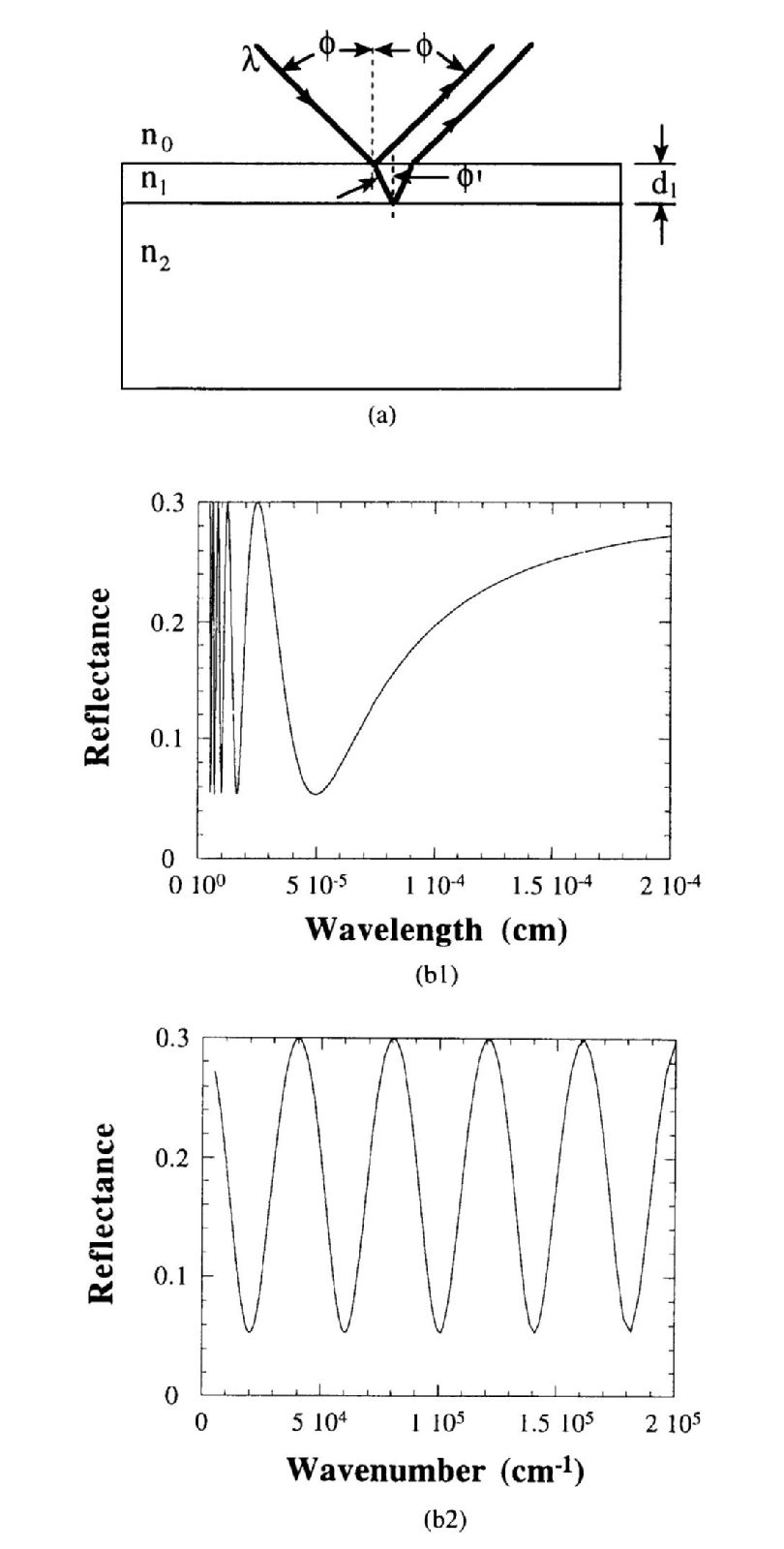# Reflection

Reflection or reflectivity measurments are usually to examine the layer thickness of insulating layer on semiconducting substrates and epitaxial semiconductor films. The reflectance R of the structure( absorbing layer of thickness d1 on a nonabsorbing ) as show in Fig. 1(a) is given by$R = \frac{r_{1}^{2}e^{\alpha d_{1} }+ r_{2}^{2}e^{-\alpha d_{1}} + 2r_{1}r_{2}cos(\varphi_{1})}{e^{\alpha d_{1} }+ r_{1}^{2}r_{2}^{2}e^{-\alpha d_{1}} + 2r_{1}r_{2}cos(\varphi_{1})}$    ---------(1)Fig.1 (a) Reflection spectroscopy schematic; (b) theoretical reflectance for SiO2 on Si.

Tox= 100 mm, n0 = 1, n1= 1.46, and n2= 3.42,$\phi =50^{0}$

Where$r_{1}=\frac{n_{0}-n_{1}}{n_{0}+n_{1}}$ ;$r_{2}=\frac{n_{1}-n_{2}}{n_{1}+n_{2}}$ ;$\varphi_{1}=\frac{4\pi n_{1}d_{1}cos(\phi^{/} ) }{\lambda }$ ;$\phi^{/}=sin^{-1}[\frac{n_{0}sin(\phi )}{n_{1}}]$

For a nonabsorbing layer,$\alpha =0$ in Eq.(1)

The reflectance exhibits maxima at the wavelengths (max)$\lambda (max)=\frac{2n_{1}d_{1}cos(\phi^{/} )}{m}$           ---------(2)

Where m = 1,2,3,…

Taking Eq.(2) at two maxima and subtracting one from the other can give the layer thickness d1$d_{1}=\frac{i}{2n_{1}( \frac{1}{\lambda_{0} }-\frac{1}{\lambda_{i}})cos(\phi^{/} )}$      ---------(3)

Where i= number of complete cycles from$\lambda_{0}$ to$\lambda_{i}$ , the two wavelength peaks that bracket the i cycles. For example in Fig. 1(b2) for the first two peaks, i= 1,$\frac{1}{\lambda_{0}}$= 1.62x105cm-1

and$\frac{1}{\lambda_{1}}$=1.22x105cm-1    or   i= 3,$\frac{1}{\lambda_{0}}$= 1.62x105cm-1 and$\frac{1}{\lambda_{3}}$= 4.2x104cm-1 is obtained a similar thickness.

[Ref.] D.K. Schroder,Semiconductor material and device characterization, 2nd edition,John Wiley & Son,1998.